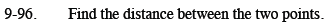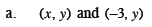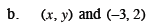Home > CCA2 > Chapter 9 > Lesson 9.3.2 > Problem9-96

9-96.
1. Find the distance between the two points. Homework Help ✎

1. (x, y) and (−3, y)

2. (x, y) and (−3, 2)Use the distance formula.

$d=\sqrt{\left(x-\left(-3\right)\right)^2+\left(y-y\right)^2}$Try this one on your own. Use the distance formula.